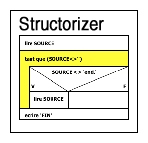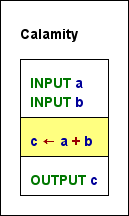S T R U C T O R I Z E R - User Guide
 Syntax > Basic Concepts

### Recursive "Definition"

An essential concept is that of an expression. An expression describes a (not necessarily numeric) value or the operations to compute a value from other values by means of functions and operators in a more or less natural way. Obvious examples of expressions are:

4 + 7
r * sin(x)
sqr(a + b)

Below there is a semi-formal recursive syntax introduction, where in some cases sort of extended Backus-Naur form is used as a well-known description format (though not quite exact here).

Atomic expressions are literals and identifiers.

### Literals

• The keywords `true` and `false` are Boolean literals.
<literal_bool> ::= true | false
• A sequence of digits possibly preceded by a sign is an integer literal:
<digit> ::= 0|1|2|3|4|5|6|7|8|9
<literal_int> ::= [+|-] <digit> {<digit>}
• A sequence of digits and letters A...F or a...f after a 0x prefix is a hexadecimal integer literal:
<hex_digit> := <digit>|A|B|C|D|E|F|a|b|c|d|e|f
<literal_hex> ::= 0x<hex_digit> {<hex_digit>}
• An integer literal followed by an 'L' character is a long integer literal.
<literal_long> ::= <literal_int>L
• A sequence of digits with a decimal point or an exponential postfix is a floating-point literal; the keyword `Infinity` and the symbol ∞ are also floating-point literals (since version 3.30-15):
<literal_float> ::= <literal_int> . <digit>{<digit>} [ E [+|-] <digit>{<digit>} ]
| [+|-] . <digit> {<digit>}[ E [+|-] <digit>{<digit>} ]
| Infinity | ∞
• A single printable character (except a single quote) enclosed in apostrophes (i.e., single quotes) is regarded as character literal:
<literal_char> ::= '<character>'
But well, certain escape notations as e.g. '\n', '\t', '\0', '\'', and '\u0123' are also valid character literals.
• Other character sequences enclosed in single or double quotes are string literals (if it's not a character literal); a string literal may also contain certain escape sequences (in particular, the enclosing delimiter, i.e. a single or double quote, respectively, must be escaped with preceding backslash if occurring within the string literal content).
<literal_string> ::= " {<character>} "  |  ' {<character>} '
• Integer, long, and floating-point literals may together be qualified as numerical literals:
<literal_num> ::= <literal_int> | <literal_hex> | <literal_long> | <literal_float>

Examples:

• true is a Boolean literal, meaning the logical value TRUE.
• -12 is an integral meaning the obvious value of minus twelve.
• 12.97 and -6.087e4 are non-integral (floating-point) numeric literals.
• '9' is not a numeric but a character literal.
• "Attention!" and 'more than 1 character' are string literals.
• "He called me \"moron\" when I left." and 'is"ok' are valid string literals, "oh"no" ist not.
• 'a' is a character literal whereas "a" is a string literal.
• ∞ is a floating-point (double) literal meaning an infinite positive value.

### Identifiers

An identifier is a name for certain concepts. In contrast to literals, identifiers require some user-specific declaration or introduction that associate them with a storage place or value.

• A dense sequence of ASCII letters, digits and underscores, ideally beginning with a letter, not at least beginning with a digit, is an identifier:
<letter> ::= A|B|C|...|Z|a|b|c|...|z
<identifier> ::= (_|<letter>){_|<letter>|<digit>}
• A sequence of identifiers, separated by dots, is called a qualified name, designating a named component of a structured object (record, struct):
<qual_name> ::= <identifier> | <qual_name> . <identifier>

The trouble with this "definition" is that the dot is actually an access operator and component access can also apply to e.g. an indexed variable. In some languages (e.g. C, C++) even the brackets for index notations are regarded as operators.

Examples:

• kill_bill is an identifier, off the record is not (there must not be spaces within).
• person.birthdate may be a qualified identifier to designate a component within a structured object. If this component is structured itself then e.g. person.birthdate.month can also be a valid qualified identifier.
• `Infinite` and `true` are not regarded as identifiers, because they are reserved as literal keywords.

### Expressions

• Literals are expressions.
<atomic_expr> ::= <literal>
<atomic_log_expr> ::= <literal_bool>
• Qualified names are valid expressions if they represent a valid access path to a nested component within a structured object the structure of which is appropriately defined by a type definition.
<atomic_expr> ::= <qual_name>
<atomic_log_expr> := <qual_name>
• An identifier followed by a pair of parentheses, which enclose a comma-separated list of expressions is a function or procedure call. Functions return a value, procedures don't. You find a list of provided built-in functions and procedures in the User Guide.
Function references are expressions, procedure references are not.
<atomic_expr> ::= <identifier> '(' <expression_list> ')'
<expression_list> ::=  | <expression_list> , <expression>
• Expressions joined by suited operator symbols are expressions. You find a table of accepted operator symbols in the Structorizer User Guide.
<factor> ::= [ + | - ] <atomic_expression>
<not_expr> ::= (not | !) <atomic_log_expr>
<mult_expr> ::= <factor> | <mult_expr> ( * | / | div | mod | % ) <factor>
<log_expr> ::= <add_expr> ( = | == | <> | < | > | <= | >= ) <add_expr> | <not_exp>
<log_and_expr> ::= <not_expr> |  <log_and_expr> ( and | && ) <log_expr>
<log_expression> ::= <log_and_expr> | <log_expression> ( or | || | xor ) <log_expression>
<cond_expr> ::= <log_expression> ? <expression> : <cond_expr>
• An expression enclosed in parentheses is an expression.
<atomic_expr> ::= '(' <expression> ')'
<atomic_log_expr> ::= '(' <log_expression> ')'
• A qualified name followed by an integer expression in brackets is an array element reference and as such an expression (if the array is defined).
<indexed_name> ::= <qual_name> '[' <expression> ']'
<atomic_expr> ::= <indexed_name>
• A comma-separated list of expressions as described above, enclosed in braces, is an array-initializing expression (only usable in assignments, as routine arguments, as input, and in FOR-IN loops).
<init_expr_a> ::= '{' <expression_list> '}'
• A defined record type identifier, followed by a pair of braces that include comma-separated triples of a declared component identifier, a colon, and an expression is a record-initializing expression.
<init_expr_r> ::= <identifier> '{' <comp_init_list> '}'
<comp_init_list> ::=  | <comp_init_list>, <comp_init>
<comp_init> ::= <identifier> : <expression>
• Since version 3.28-06, a smart record-initializing expression is supported. It still starts with a defined record type identifier, but the following pair of braces may contain a mere list of expressions as in the array-initializing expression. In this case the values will be assigned to the components in order of their declaration in the respective record type definition. There must not be more expressions in the list than components in the type (but there might be less). It is also allowed that an incomplete expression list is followed by a sequence of comma-separated triples of name, colon, and expression as before. Simple expressions following a component initialization triple, however, are ignored (examples see Records).
<comp_init> ::= [ <identifier> : ] <expression>

<expression> ::= <add_expression> | <log_expression> | <init_expr_a> | <init_expr_r> | <cond_expr>

• There are no other expressions.
• The type of an expression is derived from the used operators and operand expressions and functions. (The incorrect BNF snippets above were simply to give a vague idea how type deduction might work in a grammar-defined way. To provide a halfway exact parsable grammar would require much more non-terminal vocabulary and hundreds of BNF rules with the major weak point of undeclared variables.)
• A Boolean expression may be constructed with comparison operators or consist of operands with Boolean value etc.
• On execution, the syntax is context-sensitive, i.e. the actual variable and constant types decide whether the expression is well-formed and can be evaluated. But then its result type is unambiguous.
Consider the following diagram. Looks pretty simple and straightforward, right? Entered 5 and 7, the result will be 12, okay. But wait — what if the user enters an array or record initializer? Then the yellow expression would be completely illegal! If one of the inputs is a string then variable c would be a string, otherwise with one of a or b being false or true illegal again, with two numeric values c would become a floating point number if a or b had been entered with a decimal point, else possibly an integer result. And so on.### Statements

Statements describe some executable action. In many traditional programming languages, statements are no kind of expression, neither they are in Structorizer. They may contain and use expressions. Elements of Nassi-Shneiderman diagrams represent statements, not expressions. They may be simple (atomic: Instruction, Call, or Jump elements) or structured (i.e., they contain nested elements, any remaining kind of element).
• An assigment is a statement (see Instruction in the user guide)
( <qual_name> | <indexed_name> ) ( <- | := ) <expression>
In some programming languages (like C), assignments are expressions themselves and may thus be used as terms in more complex expressions, this does not hold in Structorizer, though.
• A procedure reference is a statement (instruction). Depending on whether the referenced procedure is a built-in one or referes to a user-defined subroutine diagram, either an Instruction element or a Call element is required to place the procedure reference.
<statement> ::= <identifier> '(' <expression_list> ')'
Further statements are:
• input statement
<statement> ::= <input> [ <literal_string> [,] ] (<qualified_name> | <indexed_name> )
• output statement
<statement> ::= <output> <expression_list>
• In a wide interpretation (e.g. C etc.), type definitions, constant definitions, and variable declarations might also be regarded as statements. In a stricter sense (e.g. Pascal), they are not. Structorizer places them in Instruction elements, so they might be subsumed under the concept "statement" here.
• Return, leave, exit, and throw statements are Jump statements.
<statement> ::= return [ <expression> ]
<statement> ::= leave [ <literal_int> ]